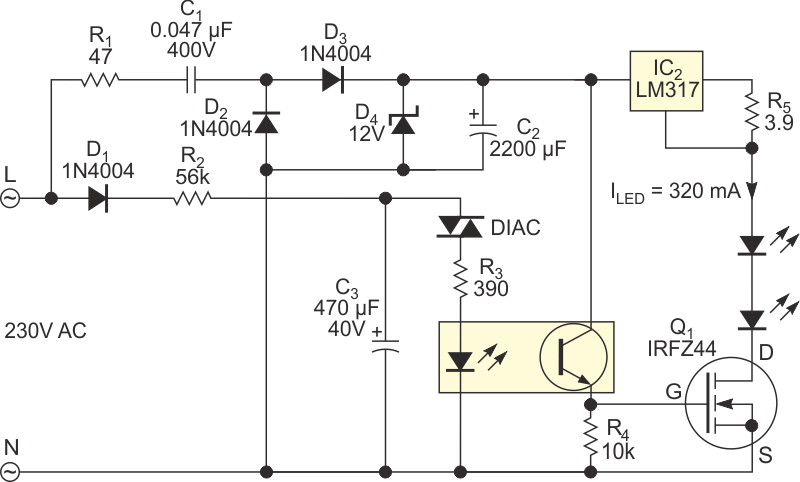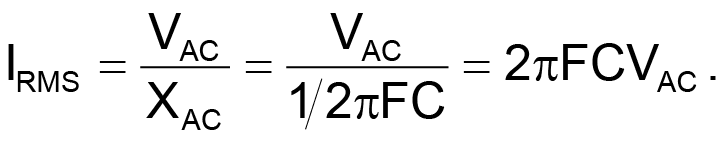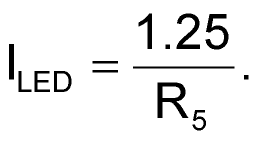# Flash an LED from ac-mains power

## Fairchild LM317

TA Babu

EDN

LED technology is opening the door to a variety of high-power-illumination applications. The circuit in Figure 1 can let you know when ac power is available. To drive a power LED from the ac line requires a converter or a similar arrangement. In this circuit, a passive dropper greatly simplifies the total design. You can also simplify the circuit to run on dc power, which lets you use it from automotive batteries to supply light at night.Figure 1. This circuit uses a simple DIAC relaxation oscillator, which activates a constant-current- switching circuit.

The design comprises an inrush-limiting resistor, R1; a half-wave rectifier with a filtering capacitor comprising D2, D3, D4, and C2; a relaxation oscillator; and two high-power LEDs. Because the circuit drives the LED with a constant current, you can use any LED color to suit the situation.

The circuit uses a simple DIAC (diode-alternating-current) relaxation oscillator, which activates a constant-current switching circuit comprising IC2 and Q1. The DIAC turns on when capacitor C3 charges through diode D1 and resistor R2 from the mains voltage. After a number of half-cycles of the mains, the voltage on C3 exceeds the break-over voltage of the DIAC, the DIAC conducts, and C3 discharges through R3 and optocoupler IC1. The optocoupler activates the constant-current switching circuit, resulting in a brief, intense flash of light from the LEDs.

High-voltage capacitor C1, part of the passive dropper, limits the current drawn from the power line, as the following equation shows:A 47 Ω metal-oxide resistor, R1, acts as an inrush-current limiter. Because the LEDs require a lot of energy, it’s not feasible to directly drive them using a small-value capacitive dropper. Instead, this circuit uses a 2200-μF capacitor, C2, to collect and store energy from the power line between flashes. Zener diode D4 limits the capacitor voltage to 12 V.

The easiest constant-current approach is to use an adjustable linear regulator, such as LM317. The regulator maintains a voltage of 1.25 V across series resistor R5. The 1.25 V is the reference voltage of the regulator. Consequently, you can determine the load current with the following equation:The active current limiting is 320 mA, which is sufficient to produce an intense light flash.

As a note of caution, this circuit has no galvanic isolation from the ac mains. Most nodes are, therefore, at mains potential and hence dangerous. You should not construct this circuit unless you have experience in handling high-voltage circuits.

EDN